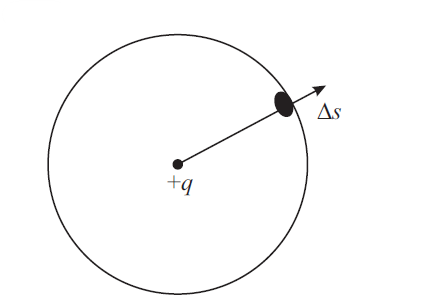# Electric Field due to a Point Charge – derivation of the formula

Last updated on May 13th, 2022 at 04:53 am

Let us apply Gauss’ Law to calculate electric field due to a point charge.
Draw a spherical surface of radius r with a point charge at the center of the sphere, as shown in Fig. 1figure 1: Electric field on a spherical surface due to a charge +q at its center

The electric field E is along the radial direction pointing away from the center and normal to the surface of the sphere at every point. The area vector is normal to the element of area Δs and is is parallel to E.

According to Gauss’ law, we can write

φE = iΣ Ei.Δsi = q/ε0 ………………. (1)

[The electric flux ΦE is defined as the scalar product of Area vector A and electric field E. Hence, ΦE = E . A = EA cos θ
Here, the area vector is normal to the element of area Δs and is is parallel to E. Hence, θ = 0°. And, cos θ = cos 0° = 1. Hence, ΦE = EA ]

E is same on all points on the surface, we can write
φ E = EA = E x 4πr²

From equation (1) we can write:
E × 4πr² = q/ε0

E = q/ (4π ε0 r² )

=>E = [1/(4πε0)] [q/r²] ………. (1) (Electric Field due to a Point Charge)

## Coulomb Force derivation from Gauss’ Law

Using Gauss’ Law we have shown above that the Electric Field due to a Point Charge q can be expressed as:

E = q/ (4π ε0 r² )

or, E = [1/(4π ε0)] [q/r²]

If there is a second charge q0 placed at a point on the surface of the sphere, the magnitude of the force on this charge would be

F = q0 × E

so that, F = q q0 / (4π ε0 r² )

Do you recognize this result? It is the expression for Coulomb’s force between two static point charges.